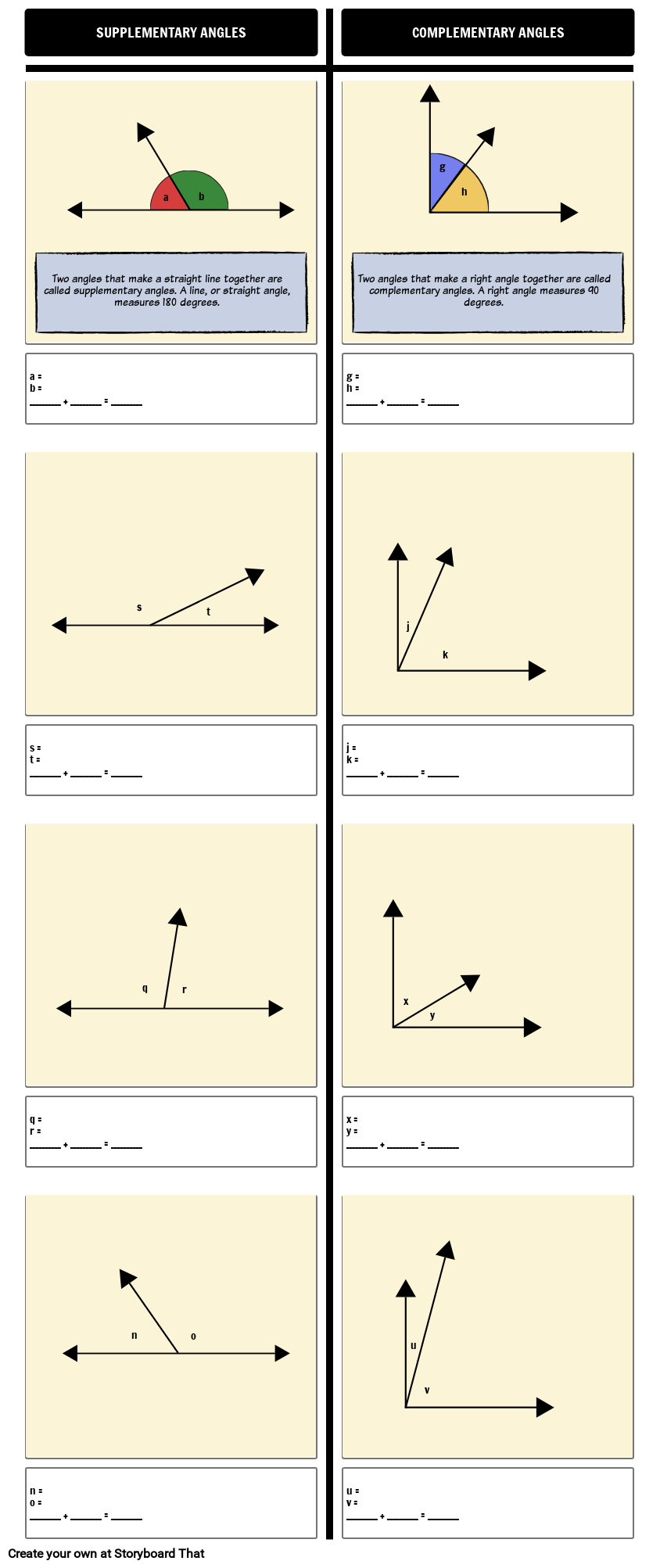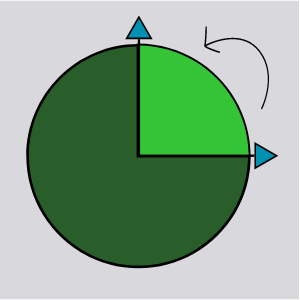# Supplementary and Complementary Angles DefinitionsYou can find this storyboard in the following articles and resources:### Introducing Angles

Lesson Plans by Anna Warfield

The basics of angles are so important for students to understand! Angles play a critical role in geometry and are the foundation for trigonometry and geometry. Students should eventually be able to read a protractor, but even just understanding if an angle is big (obtuse or reflex) or small (acute) makes a difference in elementary geometry. The following activities are a great way to provide visual aids to students and help them understand how different angles affect polygons.

### Introducing Angles

#### Storyboard Description

Supplementary and Complementary Angles Worksheet

#### Storyboard Text

• SUPPLEMENTARY ANGLES
• Two angles that make a straight line together are called supplementary angles. A line, or straight angle, measures 180 degrees.
• a
• b
• COMPLEMENTARY ANGLES
• Two angles that make a right angle together are called complementary angles. A right angle measures 90 degrees.
• g
• h
• a = b = __________ + __________ = __________
• g = h = __________ + __________ = __________
• s = t = __________ + __________ = __________
• s
• t
• j = k = __________ + __________ = __________
• j
• k
• q
• r
• x
• y
• q = r = __________ + __________ = __________
• n
• o
• x = y = __________ + __________ = __________
• u
• v
• n = o = __________ + __________ = __________
• u = v = __________ + __________ = __________
##### Over 25 Million Storyboards Created